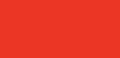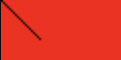# Activity 2: Modify your color board

Workshop Resources

## Example one: Make a diagonal

#This creates the original colorboard.
from PIL import Image
img = Image.new('RGB', (60, 30), 'red')
img.save('pil_red.png')
#This uses a nested while loop to change the picture.
x = 0
y = 0
while x < 10:
while y < 20:
img.putpixel( (x,y), (0, 0, 0))
x += 1
y += 1
img.save('pil_red.png')


This is the picture before adding the diagonal.This is the picture after adding the diagonal.## Example two: Make a rectangle.

#This creates the original colorboard.
from PIL import Image
img = Image.new('RGB', (60, 30), 'red')
img.save('pil_red.png')
#This uses nested for loop to change the picture.
for x in range(10, 30):
for y in range(5, 25):
img.putpixel( (x,y), (0, 0, 0))
img.save('pil_redmodified.png')


This is the picture before adding the rectangle.This is the picture after adding the rectangle.Here are two templates to assist your colorboard modifications.

#### Template One: Adding a line

# Template for adding a line
initial_position_x = 0 #make change here
initial_position_y = 0 #make change here
width = 0 #make change here
height = 0 #make change here
color = (0,0,0) #make change here
while initial_position_x < width:
while initial_position_y < height:
img.putpixel( (x,y), color)
initial_position_x += 1
initial_position_y += 1
img.save('pixel-activity2.png')


#### Template Two: Adding a rectangle

# Template for adding a rectangle
initial_position_x = 0 #make change here
initial_position_y = 0 #make change here
width = 0 #make change here
height = 0 #make change here
color = (0,0,0) #make change here
for x in range(initial_position_x, width+initial_position_x):
for y in range(initial_position_y, height+initial_position_y):
img.putpixel( (x,y), color)
img.save('pixel-activity2.png')